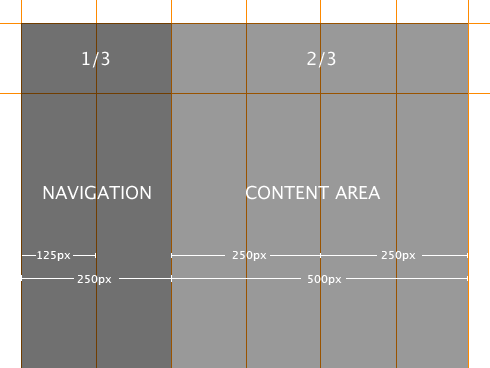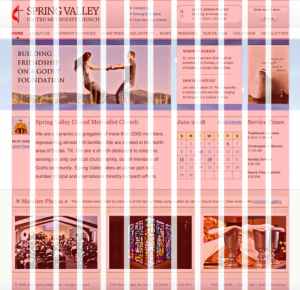`#page {    width: 70em;}`

#### 并不遥远的750#### 几个术语和一个公式`W = c * N + g * (N - 1) + 2 * m`

`W = c * N + g * (N - 1) + g = (c + g) * N`

`W = C * N`

#### 950的来历`W = N * C - g`

`950 = 12 * 80 - 10950 = 16 * 60 - 10950 = 24 * 40 - 10`#### 栅格系统的优势

1. 能大大提高网页的规范性。在栅格系统下，页面中所有组件的尺寸都是有规律的。这对于大型网站的开发和维护来说，能节约不少成本。
2. 基于栅格进行设计，可以让整个网站各个页面的布局保持一致。这能增加页面的相似度，提升用户体验。
3. 对于设计师们来说，灵活地运用栅格系统，能做出很多优秀和独特的设计。（详见《超越CSS》一书）

#### 黄金分割

`x / 1 = (1 - x) / x`

`x^2 + x - 1 = 0`

`x = (sqrt(5) - 1) / 2 ~= 0.618`960栅格，实际宽度是950. 对于 24 x 40 的情景，最接近黄金分割的两栏布局是 350 : 590, 栏数比例为 9 : 15:#### 高度方向上的栅格

`N(560) = N(2^4 * 5 * 7) = 18560 / 960 ~= 0.583`

N(560)比较大，同时可以让高宽比接近黄金分割。针对560, 我们采用 14 x 40 栅格：